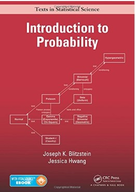Introduction To Probability - 1 Edition - Chapter 1 - Problem 41
Register Now

Join StudySoup

Get Full Access to Introduction To Probability - 1 Edition - Chapter 1 - Problem 419781466575578

# Show that for any events A and B, P(A) + P(B) 1 P(A \ B) P(A [ B) P(A) + P(B). For each

Introduction to Probability | 1st Edition

Problem 41

Show that for any events A and B, P(A) + P(B) 1 P(A \ B) P(A [ B) P(A) + P(B). For each of these three inequalities, give a simple criterion for when the inequality is actually an equality (e.g., give a simple condition such that P(A \ B) = P(A [ B) if and only if the condition hol

Accepted Solution
Step-by-Step Solution:
Step 1 of 3

Chapter 14 Scatterplot: Relationship between 2 quantitative variable measured by the same individual. X axis: explanatory variable/ independent variable (Think I the scientist can control) Y axis: response variable/ dependent  Example: The growth of a plant (inches) and the amount of sunlight given (seconds).  Seconds of sunlight is the Independent variable ( something that can be controlled)  Inches the plant grows would be the Dependent variable ( depends on the amount of light given)  Sunlight (seconds) should be on the x axis Interpreting Scatterplots  Look at overall pattern  Look for outliers  Described by form, direction and strength Form: clustered, curved, linear Strength: How close points follow the form

###### Chapter 1, Problem 41 is Solved

Step 2 of 3

Step 3 of 3

Unlock Textbook Solution